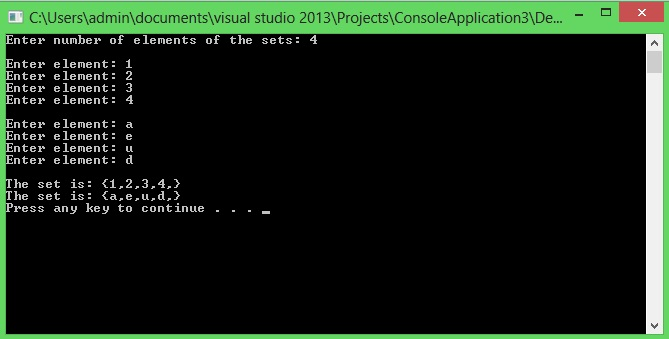# Template class

In this part you will learn: 1. What is template class 2. Why we need template class 3. Syntax 4. Program 5. Output Template Class Just like template functions, we can declare a class template too. For those who don’t know what template function is, it is functionality in c++ in which we generalize the data type so that it can be used for any data type as per the values using the template function. In case of classes, it can differ by the type(int, char, double etc) of object we are making for a class, then all the member function will start treating the data members as that type. It allows classes to have members that use template parameters as types. Many programming tasks, we require a class which can word for all data types, So instead of defining every data type separately, we generalize the class using Template class functionality. Syntax Following is the syntax of defining a member function outside the class. template class Class_Name { any_name variable_names; } template void Class_name :: function_name(parameters) { //definiton } In the above syntax, the most important thing to note is that while defining a function outside the class, first we have to define that it is a template thing, then while referring to the class we have to type the name of variable we are generalizing the class with. Program with Template Class The following example illustrates the concept of Template Class by the usage of Sets. Basic Step:
`#include<iostream>#include<conio.h>using namespace std;`
These two are the most common and basic lines of a c++ program. The first one iostream is used to grant us most of the basic functions of C++ like Input and Output functions. The second one conio.h means console input output, the black screen we see is the console. Using namespace std; provides us an ease of using all the library functions without specifying that this function belongs to standard library. Without including it, we have to write ‘std’ with all the built-in functions. Class
`template <typename T>class set{public:	T *ele;	int size; 	set(){		size = 0;		ele = NULL;	} 	set(int n){		size = n;		ele = new T[size];	} 	~set(){			if (size > 0)			delete[]ele;		ele = NULL;	} 	void fill_set();	void print_set();}; template <typename T>void set<T>::fill_set(){	cout << endl;	for (int i = 0; i < size; i++)		{		cout << "Enter element: ";		cin >> ele[i];		}} template <typename T>void set<T>::print_set(){	cout << endl << "The set is: {";	for (int i = 0; i < size; i++)		{		cout << ele[i];		cout << ",";		}	cout << "}";}`
In the above class which is a template class, I have declared the member variable which is a pointer of type T, so that whatever type the user gives while initializing an object, the member variable ele gets that type. Furthermore I have defined the default and overloaded constructor as well as destructor in the class and the functions fill_set and print_set outside the class so you get an idea how to define them in both ways. One more important thing here is while dynamically allocating the memory in the overloaded constructor, I have written “ele=new T[size]” the reason behind this is that the memory going to be allocated dynamically will be depended on the type of object we are initializing. i.e. char and int have different types of memory allocation so we have generalized it with T. Let us now take a look at the main (driver) function of the program.
`int main(){	int size; 	cout << "Enter number of elements of the sets: ";	cin >> size; 	set<int> set1(size);	set<char> set2(size); 	set1.fill_set();	set2.fill_set(); 	set1.print_set();	set2.print_set(); 	cout << endl; 	system("pause");}`
In the main function first user is being asked about the size of the sets, later two objects are being initialized of size “size”. The key point here is that “set set1(size);” means that we are initializing a class with int as the type of the elements we want to store in the set. It can be any other data type like float, double, char etc. Then the functions of fill_set and print_set are being called to store and print the elements of set on the screen respectively. Output